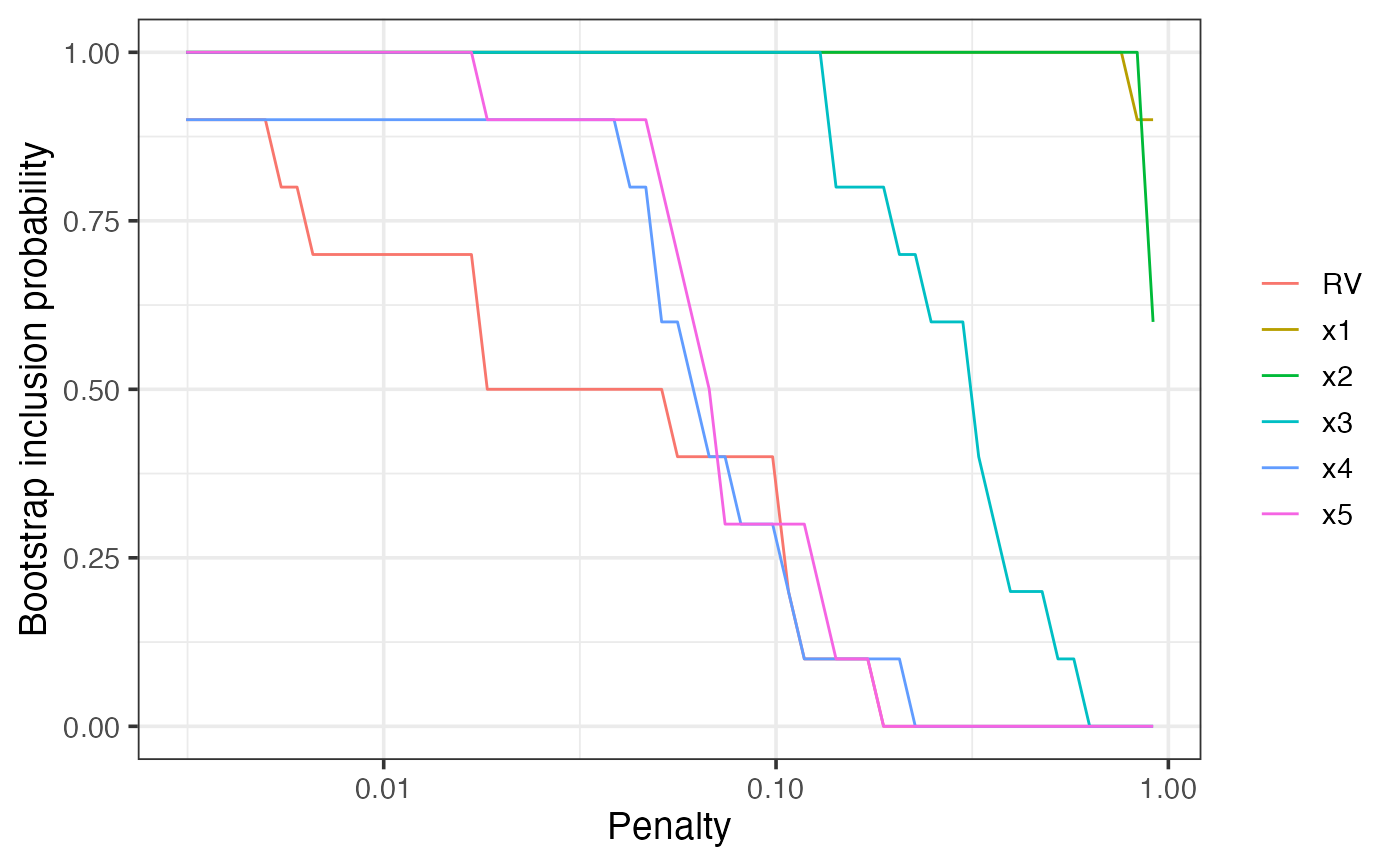Model stability and variable importance plots for glmnet

bglmnet(
mf,
nlambda = 100,
lambda = NULL,
B = 100,
penalty.factor,
screen = FALSE,
redundant = TRUE,
cores = NULL,
force.in = NULL,
seed = NULL
)

## Arguments

mf a fitted 'full' model, the result of a call to lm or glm. how many penalty values to consider. Default = 100. manually specify the penalty values (optional). number of bootstrap replications Separate penalty factors can be applied to each coefficient. This is a number that multiplies lambda to allow differential shrinkage. Can be 0 for some variables, which implies no shrinkage, and that variable is always included in the model. Default is 1 for all variables (and implicitly infinity for variables listed in exclude). Note: the penalty factors are internally rescaled to sum to nvars, and the lambda sequence will reflect this change. logical, whether or not to perform an initial screen for outliers. Highly experimental, use at own risk. Default = FALSE. logical, whether or not to add a redundant variable. Default = TRUE. number of cores to be used when parallel processing the bootstrap (Not yet implemented.) the names of variables that should be forced into all estimated models. (Not yet implemented.) random seed for reproducible results

## Details

The result of this function is essentially just a list. The supplied plot method provides a way to visualise the results.

plot.bglmnet

## Examples

n = 100
set.seed(11)
e = rnorm(n)
x1 = rnorm(n)
x2 = rnorm(n)
x3 = x1^2
x4 = x2^2
x5 = x1*x2
y = 1 + x1 + x2 + e
dat = data.frame(y, x1, x2, x3, x4, x5)
lm1 = lm(y ~ ., data = dat)
# \dontshow{
bg1 = bglmnet(lm1, seed = 1, B=10)
plot(bg1)# }
if (FALSE) {
bg1 = bglmnet(lm1, seed = 1)
# plot(bg1, which = "boot_size", interactive = TRUE)
plot(bg1, which = "boot_size", interactive = FALSE)
# plot(bg1, which = "vip", interactive = TRUE)
plot(bg1, which = "vip", interactive = FALSE)
}